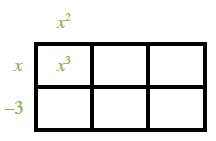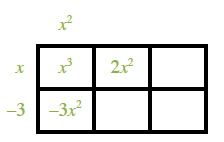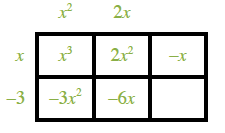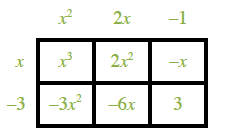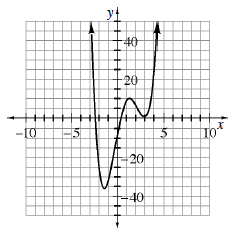### Home > INT3 > Chapter 8 > Lesson 8.3.2 > Problem8-112

8-112.

Carlo is trying to factor the polynomial $p\left(x\right) = x^{4} – 4x^{3} – 4x^{2} + 24x – 9$ to determine all of its zeros. He discovers one factor by making a guess and dividing the polynomial, so he has $p\left(x\right) = \left(x – 3\right)\left(x^{3} – x^{2} – 7x + 3\right)$. Now he is trying to factor $x^{3} – x^{2} – 7x + 3$, so he tries dividing it by $\left(x + 3\right),$ then by $\left(x + 1\right)$, and finally by $\left(x – 1\right)$, but none works without a remainder. Then Teo comes by and says, “You should look at the graph.”

1. How does the graph help?

It shows that $x = 3$ is a double root, so $\left(x − 3\right)$ is a repeated factor.

2. Complete the problem.

Divide $x^{3} − x^{2} − 7x + 3$ by $\left(x − 3\right).$2 by 3 rectangle, labeled as follows: left edge, x, minus 3, top edge, left, x, squared, interior top, left, x squared.

Labels added: Interior bottom, left, negative 3, x, squared, interior top, middle, 2, x squared.

Labels added: top edge, middle, 2, x, interior bottom, middle, negative 6, x, interior top, right, negative x.

Labels added: top edge, right, negative 1, interior bottom, right, 3.

Now use the Quadratic Formula to solve for $x$.

$x = 3, -1 \pm \sqrt{2}$